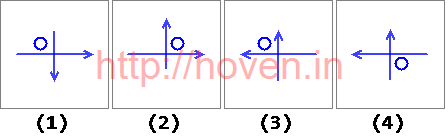# Image Series Quiz Set 008

### Question 1

Which image would be the next image if the pattern is continued?

Question Image:A

1.

B

2.

C

3.

D

4.

Soln.
Ans: c
```1   2   3
=========
4   5   6
=========
7   8   9
```

With reference to the above diagram, on each step the movements are as shown below.

1. 1 moves to 2
2. 2 moves to 3
3. 3 moves to 4
4. 4 moves to 5
5. 5 moves to 6
6. 6 moves to 7
7. 7 moves to 8
8. 8 moves to 9
9. 9 moves to 1

The icons are cyclically shifting.

### Question 2

Which image would be the next image if the pattern is continued?

Question Image:A

1.

B

2.

C

3.

D

4.

Soln.
Ans: c

This is a clock with one long arm and the other is short arm. The long arm moves by 90° whereas the short arm is moving by 45°

### Question 3

Which image would be the next image if the pattern is continued?

Question Image:A

1.

B

2.

C

3.

D

4.

Soln.
Ans: a

Bubble is moving clockwise. Arrows are flipping alternatively. Once the vertical arrow flips, the next time the horizontal arrow flips. So in the answerm the bubble should be in the lower right corner, the vertical arrow should be down and the horizontal arrow should be towards the right.

### Question 4

Which image would be the next image if the pattern is continued?

Question Image:A

1.

B

2.

C

3.

D

4.

Soln.
Ans: c

Ignore everything else and Observe the middle row. First a black circle disappears, then a square appears in its place. When a square appears, an arrow also appears at the bottom.

### Question 5

Which image would be the next image if the pattern is continued?

Question Image:A

1.

B

2.

C

3.

D

4.

Soln.
Ans: d

Horizontal lines increase from one to three and then repeat from 1 onwards again. The arrows change direction alternately. In the answer figure, we will have two horizontal lines.